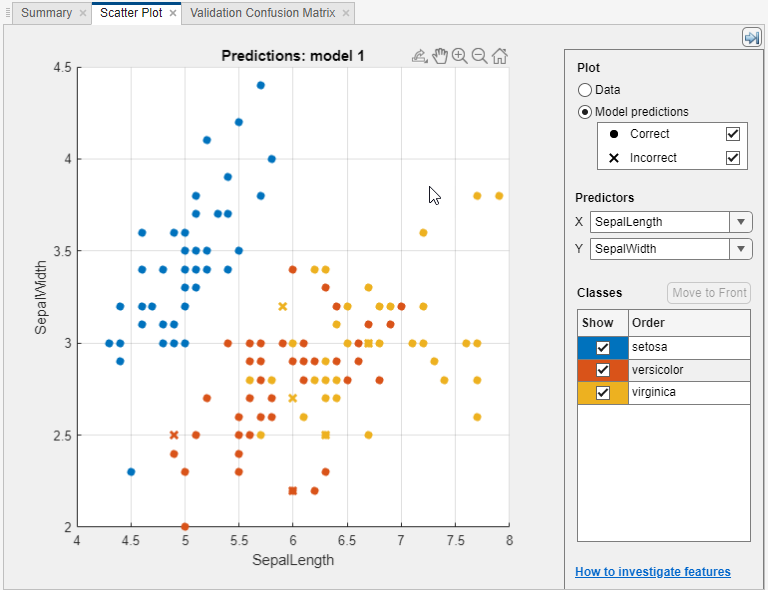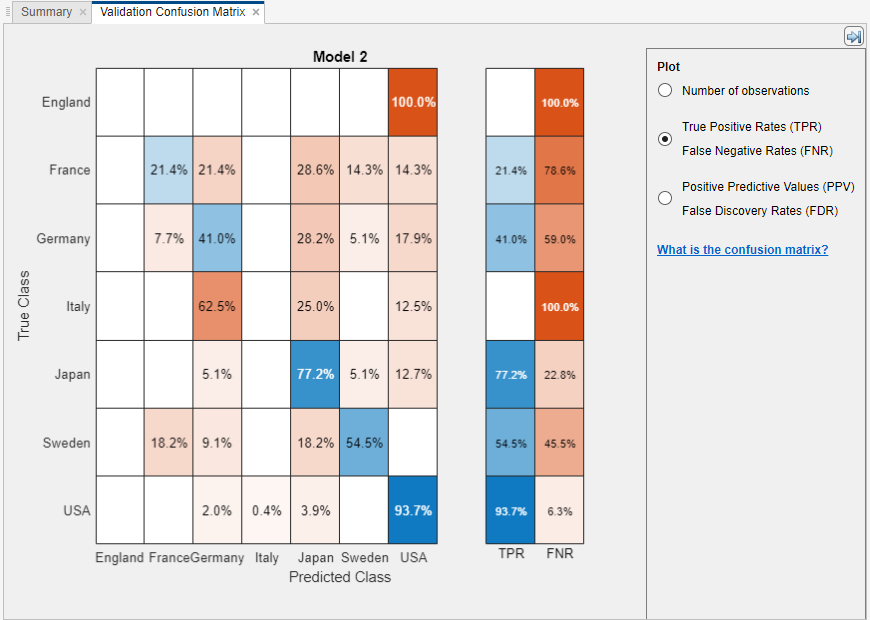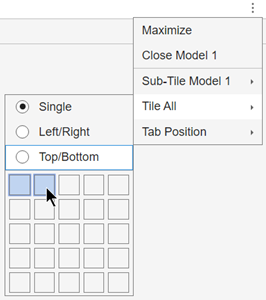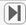## Assess Classifier Performance in Classification Learner

After training classifiers in Classification Learner, you can compare models based on accuracy scores, visualize results by plotting class predictions, and check performance using the confusion matrix and ROC curve.

• If you use k-fold cross-validation, then the app computes the accuracy scores using the observations in the k validation folds and reports the average cross-validation error. It also makes predictions on the observations in these validation folds and computes the confusion matrix and ROC curve based on these predictions.

Note

When you import data into the app, if you accept the defaults, the app automatically uses cross-validation. To learn more, see Choose Validation Scheme.

• If you use holdout validation, the app computes the accuracy scores using the observations in the validation fold and makes predictions on these observations. The app also computes the confusion matrix and ROC curve based on these predictions.

• If you use resubstitution validation, the score is the resubstitution accuracy based on all the training data, and the predictions are resubstitution predictions.

### Check Performance in the Models Pane

After training a model in Classification Learner, check the Models pane to see which model has the best overall accuracy in percent. The best Accuracy (Validation) score is highlighted in a box. This score is the validation accuracy. The validation accuracy score estimates a model's performance on new data compared to the training data. Use the score to help you choose the best model.

• For cross-validation, the score is the accuracy on all observations not set aside for testing, counting each observation when it was in a holdout (validation) fold.

• For holdout validation, the score is the accuracy on the held-out observations.

• For resubstitution validation, the score is the resubstitution accuracy against all the training data observations.

The best overall score might not be the best model for your goal. A model with a slightly lower overall accuracy might be the best classifier for your goal. For example, false positives in a particular class might be important to you. You might want to exclude some predictors where data collection is expensive or difficult.

To find out how the classifier performed in each class, examine the confusion matrix.

### View and Compare Model Metrics

You can view model metrics in the model Summary tab and use these metrics to assess and compare models. The Training Results metrics are calculated on the validation set. The Test Results metrics, if displayed, are calculated on an imported test set. For more information, see Evaluate Test Set Model Performance.Model Metrics

MetricDescriptionTip
AccuracyPercentage of observations that are correctly classifiedLook for larger accuracy values.
Total costTotal misclassification costLook for smaller total cost values. Make sure the accuracy value is still large.

You can sort models based on the different model metrics. To select a metric for model sorting, use the Sort by list at the top of the Models pane.

You can also delete unwanted models listed in the Models pane. Select the model you want to delete and click the button in the upper right of the pane, click the button in the Models section of the Classification Learner tab, or right-click the model and select Delete. You cannot delete the last remaining model in the Models pane.

### Plot Classifier Results

Use a scatter plot to examine the classifier results. To view the scatter plot for a model, select the model in the Models pane. On the Classification Learner tab, in the Plots section, click the arrow to open the gallery, and then click in the Validation Results group. After you train a classifier, the scatter plot switches from displaying the data to showing model predictions. If you are using holdout or cross-validation, then these predictions are the predictions on the held-out (validation) observations. In other words, the software obtains each prediction by using a model that was trained without the corresponding observation.

To investigate your results, use the controls on the right. You can:

• Choose whether to plot model predictions or the data alone.

• Show or hide correct or incorrect results using the check boxes under Model predictions.

• Choose features to plot using the X and Y lists under Predictors.

• Visualize results by class by showing or hiding specific classes using the check boxes under Show.

• Change the stacking order of the plotted classes by selecting a class under Classes and then clicking Move to Front.

• Zoom in and out, or pan across the plot. To enable zooming or panning, place the mouse over the scatter plot and click the corresponding button on the toolbar that appears above the top right of the plot.To export the scatter plots you create in the app to figures, see Export Plots in Classification Learner App.

### Check Performance Per Class in the Confusion Matrix

Use the confusion matrix plot to understand how the currently selected classifier performed in each class. After you train a classification model, the app automatically opens the confusion matrix for that model. If you train an "All" model, the app opens the confusion matrix for the first model only. To view the confusion matrix for another model, select the model in the Models pane. On the Classification Learner tab, in the Plots section, click the arrow to open the gallery, and then click Confusion Matrix (Validation) in the Validation Results group. The confusion matrix helps you identify the areas where the classifier performed poorly.

When you open the plot, the rows show the true class, and the columns show the predicted class. If you are using holdout or cross-validation, then the confusion matrix is calculated using the predictions on the held-out (validation) observations. The diagonal cells show where the true class and predicted class match. If these diagonal cells are blue, the classifier has classified observations of this true class correctly.

The default view shows the number of observations in each cell.

To see how the classifier performed per class, under Plot, select the True Positive Rates (TPR), False Negative Rates (FNR) option. The TPR is the proportion of correctly classified observations per true class. The FNR is the proportion of incorrectly classified observations per true class. The plot shows summaries per true class in the last two columns on the right.

Tip

Look for areas where the classifier performed poorly by examining cells off the diagonal that display high percentages and are orange. The higher the percentage, the darker the hue of the cell color. In these orange cells, the true class and the predicted class do not match. The data points are misclassified.In this example, which uses the `carbig` data set, the fifth row from the top shows all cars with the true class Japan. The columns show the predicted classes. Of the cars from Japan, 77.2% are correctly classified, so 77.2% is the true positive rate for correctly classified points in this class, shown in the blue cell in the TPR column.

The other cars in the Japan row are misclassified: 5.1% of the cars are incorrectly classified as from Germany, 5.1% are classified as from Sweden, and 12.7% are classified as from the USA. The false negative rate for incorrectly classified points in this class is 22.8%, shown in the orange cell in the FNR column.

If you want to see numbers of observations (cars, in this example) instead of percentages, under Plot, select Number of observations.

If false positives are important in your classification problem, plot results per predicted class (instead of true class) to investigate false discovery rates. To see results per predicted class, under Plot, select the Positive Predictive Values (PPV), False Discovery Rates (FDR) option. The PPV is the proportion of correctly classified observations per predicted class. The FDR is the proportion of incorrectly classified observations per predicted class. With this option selected, the confusion matrix now includes summary rows below the table. Positive predictive values are shown in blue for the correctly predicted points in each class, and false discovery rates are shown in orange for the incorrectly predicted points in each class.

If you decide there are too many misclassified points in the classes of interest, try changing classifier settings or feature selection to search for a better model.

To export the confusion matrix plots you create in the app to figures, see Export Plots in Classification Learner App.

### Check the ROC Curve

View the receiver operating characteristic (ROC) curve after training a model. In the Plots section, click the arrow to open the gallery, and then click ROC Curve (Validation) in the Validation Results group. The ROC curve shows the true positive rate (TPR) versus the false positive rate (FPR) for different thresholds of classification scores computed by the current trained classifier.

The marker on the plot shows the values of the false positive rate and the true positive rate for the current classifier. For example, a false positive rate of 0.2 indicates that the current classifier incorrectly assigns 20% of the negative class observations to the positive class. A true positive rate of 0.9 indicates that the classifier correctly assigns 90% of the positive class observations to the positive class.

For a multiclass classifier, you can select different classes to plot in the app. The app formulates a set of one-versus-all binary classification problems to have one binary problem for each class, and finds a ROC curve for each class using the corresponding binary problem. Each binary problem assumes that one class is positive and the rest are negative. The marker on the plot shows the performance of the current classifier for each class in its one-versus-all binary problem.

The Area Under Curve value (area under a ROC curve, or AUC) corresponds to the integral of a ROC curve (TPR values) with respect to FPR from `FPR` = `0` to `FPR` = `1`. The AUC value is a measure of the overall quality of the classifier. The AUC values are in the range from `0` to `1`, and larger AUC values indicate better classifier performance. Compare classes and trained models to see if they perform differently in the ROC curve.

For more information, see `perfcurve`, Performance Curves by perfcurve, and Introduction to ROC Curve.

To export the ROC curve plots you create in the app to figures, see Export Plots in Classification Learner App.

### Compare Model Plots by Changing Layout

Visualize the results of models trained in Classification Learner by using the plot options in the Plots section of the Classification Learner tab. You can rearrange the layout of the plots to compare results across multiple models: use the options in the Layout button, drag and drop plots, or select the options provided by the Document Actions arrow located to the right of the model plot tabs.

For example, after training two models in Classification Learner, display a plot for each model and change the plot layout to compare the plots by using one of these procedures:

• In the Plots section, click and select Compare models.

• Click the second model tab name, and then drag and drop the second model tab to the right.

• Click the Document Actions arrow located to the far right of the model plot tabs. Select the `Tile All` option and specify a 1-by-2 layout.Note that you can click the Hide plot options buttonat the top right of the plots to make more room for the plots.

### Evaluate Test Set Model Performance

After training a model in Classification Learner, you can evaluate the model performance on a test set in the app. This process allows you to check whether the validation accuracy provides a good estimate for the model performance on new data.

1. Import a test data set into Classification Learner. Alternatively, reserve some data for testing when importing data into the app (see (optional) Reserve Data for Testing).

• If the test data set is in the MATLAB® workspace, then in the Test section on the Classification Learner tab, click and select From Workspace.

• If the test data set is in a file, then in the Test section, click and select From File. Select a file type in the list, such as a spreadsheet, text file, or comma-separated values (`.csv`) file, or select All Files to browse for other file types such as `.dat`.

In the Import Test Data dialog box, select the test data set from the Test Data Set Variable list. The test set must have the same variables as the predictors imported for training and validation. The unique values in the test response variable must be a subset of the classes in the full response variable.

2. Compute the test set metrics.

• To compute test metrics for a single model, select the trained model in the Models pane. On the Classification Learner tab, in the Test section, click and select Test Selected.

• To compute test metrics for all trained models, click and select Test All in the Test section.

The app computes the test set performance of each model trained on the full data set, including training and validation data (but excluding test data).

3. Compare the validation accuracy with the test accuracy.

In the model Summary tab, the app displays the validation metrics and test metrics under the Training Results section and Test Results section, respectively. You can check if the validation accuracy gives a good estimate for the test accuracy.

You can also visualize the test results using plots.

• Display a confusion matrix. In the Plots section on the Classification Learner tab, click the arrow to open the gallery, and then click in the Test Results group.

• Display an ROC curve. In the Plots section, click the arrow to open the gallery, and then click in the Test Results group.

For an example, see Check Classifier Performance Using Test Set in Classification Learner App. For an example that uses test set metrics in a hyperparameter optimization workflow, see Train Classifier Using Hyperparameter Optimization in Classification Learner App.